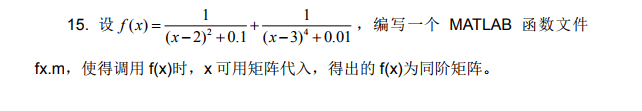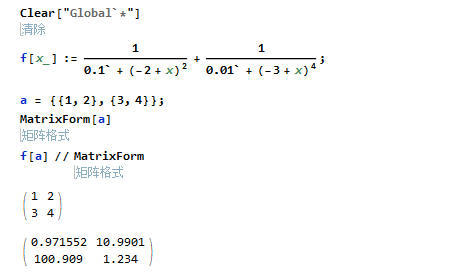Mathematica入门习题–函数的编写

• A+

第十五题Clear["Global`*"]
f[x_] := 1/(0.1` + (-2 + x)^2) + 1/(0.01` + (-3 + x)^4);
a = {{1, 2}, {3, 4}};
MatrixForm[a]
f[a] // MatrixForm

Mathematica的函数带入的数据类型不是固定的，如这里即可以带入矩阵也可以只是普通的数• 微信公众号
• 关注微信公众号
•• QQ群
• 我们的QQ群号
•